Facebook 开源 JavaScript 代码优化工具 Prepack

CIIS 2019丨CIIS 2019丨曲道奎：进化与繁衍中的智能机器人>>>• 抽象语法树（AST）Prepack运行在AST级别，使用Babel解析并生成JavaScript源代码。

• 具体执行（Concrete Execution）Prepack的核心是一个JavaScript解释器，它与ECMAScript 5几乎完全兼容，而且紧密地保持与ECMAScript 2016语言规范的一致性，你可以将Prepack中的解释器视为完全参照JavaScript实现的。解释器能够跟踪并撤销包括所有对象Mutation在内的结果，从而能够进行推测优化（Speculative Optimization）。

• 符号执行（Symbolic Execution）除了对具体值进行计算外，Prepack的解释器还可以操作受环境相互作用影响的抽象值。例如Date.now可以返回一个抽象值，你可以通过helper辅助函数（如__abstract()）手动注入抽象值。Prepack会跟踪所有在抽象值上执行的操作，在遇到分支时，Prepack会执行并探索所有可能性。所以，Prepack实现了一套JavaScript的符号执行引擎。

• 抽象释义（Abstract Interpretation）符号执行在遇到抽象值的分支时会分叉（fork），Prepack会在控制流合并点加入分歧执行（Diverged Execution）来实现抽象释义的形式。连接变量和堆属性可能会得到条件抽象值，Prepack会跟踪有关抽象值和型域（Type Domain）的信息。

• 堆序列化（Heap Serialization）当全局代码返回，初始化阶段结束时，Prepack捕获最终的堆并按顺序排列堆栈，生成直观的JavaScript新代码，创建并链接初始化堆中可访问的所有对象。堆中的一些值可能是抽象值的计算结果，对于这些值，Prepack将生成原始程序完成计算所执行的代码。

/* Hello World */
// Input
(function () {
function hello() { return 'hello'; }
function world() { return 'world'; }
global.s = hello() + ' ' + world();
})();
// Output
(function () {
s = "hello world";
})();
/* 消除抽象税 */
// Input
(function () {
var self = this;
['A', 'B', 42].forEach(function(x) {
var name = '_' + x.toString().toLowerCase();
var y = parseInt(x);
self[name] = y ? y : x;
});
})();
// Output
(function () {
_a = "A";
_b = "B";
_4 = 42;
})();
/* 斐波那契 */
// Input
(function () {
function fibonacci(x) {
return x <= 1 ? x : fibonacci(x - 1) + fibonacci(x - 2);
}
global.x = fibonacci(23);
})();
// Output
(function () {
x = 28657;
})();
/* 模块初始化 */
// Input
(function () {
let moduleTable = {};
function define(id, f) { moduleTable[id] = f; }
function require(id) {
let x = moduleTable[id];
return x instanceof Function ? (moduleTable[id] = x()) : x;
}
global.require = require;
define("one", function() { return 1; });
define("two", function() { return require("one") + require("one"); });
define("three", function() { return require("two") + require("one"); });
define("four", function() { return require("three") + require("one"); });
})();
three = require("three");
// Output
(function () {
function _2() {
return 3 + 1;
}
var _1 = {
one: 1,
two: 2,
three: 3,
four: _2
};
function _0(id) {
let x = _1[id];
return x instanceof Function ? _1[id] = x() : x;
}
require = _0;
three = 3;
})();
/* 环境相互作用与分支 */
// Input
(function(){
function fib(x) { return x <= 1 ? x : fib(x - 1) + fib(x - 2); }
let x = Date.now();
if (x === 0) x = fib(10);
global.result = x;
})();
// Output
(function () {
var _0 = Date.now();
if (typeof _0 !== "number") {
throw new Error("Prepack model invariant violation");
}
result = _0 === 0 ? 55 : _0;
})();

Prepack团队对未来的规划如下：

• 稳定现有功能集，用于预优化(Prepack）React Native代码包

• 集成React Native工具链

• 根据React Native所用模块系统的假设来构建优化

• 进一步优化序列化（Serialization），包括：消除不暴露特性（identity）的对象；消除未使用的导出属性，等等

• 预优化每个函数、基本代码块、语句、表达式

• 与ES6保持完全一致

• 支持广泛的模块系统

• 假设ES6支持某些功能，延迟完成或直接忽略Polyfill应用

• 进一步实现Web和Node.js环境中的兼容性目标

• 深入集成JavaScript虚拟机，改进堆反序列化过程，包括 ：暴露“对象懒初始化”的概念 - 以一种JavaScript无感知的方式，在首次使用对象时对其进行初始化；通过专门的字节码提高普通对象创建的编码效率；将代码分为两个阶段：1) 非环境依赖阶段，虚拟机可以安全地捕获并恢复生成的堆；2)环境依赖阶段，通过从环境中获得的值执行所有剩余的计算过程来拼凑具体的堆，等等

• 总结循环和递归

• JavaScript Playground - 通过调整JavaScript引擎体验JavaScript特性，这些引擎由JavaScript所编写，托管在浏览器中；你可以把它想象成一个“Babel虚拟机”，实现了不能被编译的JavaScript新特性

• 捉Bug - 发现异常崩溃、执行问题……

• 效果分析，例如检测模块工厂函数可能的副作用或强制纯净注释

• 类型分析

• 信息流分析

• 调用图推理，允许内联和代码索引

• 自动测试生成，利用符号执行的特性与约束求解器（Constraint Solver）结合来计算执行不同执行路径的输入

• 智能模糊(Smart Fuzzing）

• JavaScript沙盒 - 以不可观察的方式有效地测试JavaScript代码

精彩评论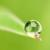Prepack：不，是你的代码！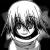引用来自“无著方知尘亦珍”的评论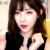最新评论（17）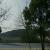引用来自“无著方知尘亦珍”的评论引用来自“dolloyo”的评论引用来自“无著方知尘亦珍”的评论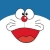引用来自“无著方知尘亦珍”的评论